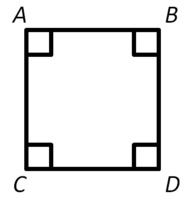100

4 x 8=

32

100

A shape with four equal sides at 90 degree angles

A square100

Liam had read that oranges are good for him. He went to buy 8 oranges at the grocery store. Each orange costs 50 cents. How much did Liam have to pay?

\$ 4.00

100

10 x 10=

100

100

4 times 10

What is 40

200

7 x 9=

63

200

The area of a square with a side length of 12 in.

144 sq. in.

200

Liam was sick last Friday.

Liam went to the doctor. There are 8 patients waiting. The nurse told him it will be his turn after 2 hours. How many minutes will it take for the doctor to see Liam?

120 minutes

200

200 x 2 =

400

200

Sum

300

11 x 12=

132

300

The perimeter of a regular hexagon with a side length of 9 cm.

54 cm2

300

Kaden usually gets up at 7:30 a.m. Since he was sick, he got up 1 hour and 30 minutes later than usual. What time did he get up?

9:00 am

300

20 + 20 + 20 + 20 + 1=

81

300

How many minutes are in 1 hour?

What is 60 minutes?

400

13 x 5 =

65

400

The measurement of a straight angle.

180 degrees

400

Liam ate 1 1/2 of an orange before he took his medicine and ate another 2 1/2 of an orange afterwards. How much oranges did he eat?

4 oranges

400

4 times 15

60

400

What is the answer to a multiplication problem?

What is the product?

500

20 x 11=

220

500

The name of a figure with 6 equal sides is call a .......

hexagon

500

Liam has some medicine at home for his headache and fever. There are 36 tablets left in the bottle and he is supposed to take 3 tablets a day. How many tablets will be left after day 11?

3 tablets

500

1001- 901=

100

500

Quotient

What is the answer to a division problem?

Click to zoom